Previous Year Questions Answers: Structure of the Atom

# Previous Year Questions Answers: Structure of the Atom - Science Class 9

Q.1. (a) What are isotopes? Write two isotopes of carbon.
(b) Write two applications of isotopes.    [NCT 2019]
Ans. (a) Atoms of the same elements containing the same number of protons but a different number of neutrons are called isotopes. Isotopes have similar chemical properties but different physical properties.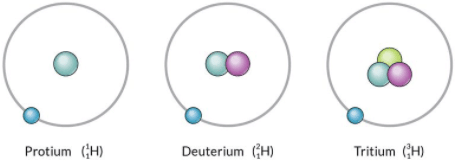Isotopes of HydrogenIsotopes of carbon: Carbon has two isotopes, carbon-12 and carbon-14.
Carbon-12 (126C)
No. of protons = 6
No. of neutrons = 6
Carbon-14 (146C)
No. of protons = 6
No. of neutrons = 8
(b) Applications:
1. An isotope of uranium is used as a fuel in nuclear reactors.
2. An isotope of cobalt is used in the treatment of cancer.

Q.2. Two elements X and Y have atomic number 16 and 19 respectively. Compare their valency, number of protons in their ions and number of electrons in their ions.    [NCT 2018]
Ans.
Element X is sulphur.

Element Y is potassium.
Valency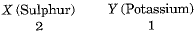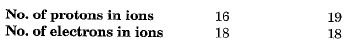Q.3. An element X forms the following compounds with hydrogen, carbon and phosphorous: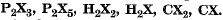Find the valencies of X and another element present in the compound.    [CBSE 2016]
Ans.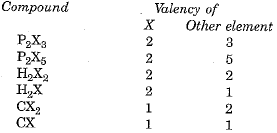Q.4. Write the electronic configuration and valency of the following:
(i) Chlorine
(ii) Sodium and
(iii) Silicon.    [CBSE 2016]
Ans.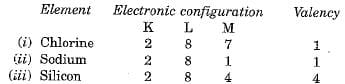Q.5. (a) Both helium and beryllium have two electrons in the valence shells. Helium is a noble gas where as Beryllium is a metal, justify.
(b) Hydrogen exists in three isotopic forms. Why are the isotopes of hydrogen chemically a like?    [CBSE 2016]
Ans.
(a) Maximum capacity of the first orbit is 2 electrons. Therefore, helium having two electrons in the valence shell is stable and hence has no tendency to react.
Beryllium has two shells K and L. The maximum capacity of the L-shell is 8 whereas beryllium has only 2 electrons in the L-shell. It will therefore react with other beryllium atoms to form Be-Be bonds. It is therefore a metal.
(b) The three isotopes of hydrogen are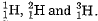The chemical property of an element depends upon the number of protons. Since the number of protons in the isotopes is the same, the isotopes are chemically alike.

Q.6. An element is represented as 168X. Find
(а) the number of electrons in elements X.
(b) a mass number of the element X.
(c) the number of neutrons in element X.    [CBSE 2016]
Ans.
The notation 168X illustrates that the element has atomic number as 8 and mass number as 16. This means
Number of protons (or electrons) = 8
Number of protons + Number of neutrons = 16
or
8 + Number of neutrons = 16
or
Number of neutrons = 16 - 8 = 8
Hence,
(a) Number of electrons in the element X = 8
(b) Mass number of element X = 16
(c) Number of neutrons in the element X = 8

Q.7. What was the drawback of Rutherford’s model of the atom?    [CBSE 2016]
Ans. It could not explain the stability of atom.

Q.8. According to Bohr and Bury scheme what is the maximum number of electrons present in M-shell of an atom?    [CBSE 2016]
Ans.
The maximum number of electrons in M-shell = 2 x 32 = 2 x 9 = 18.

Q.9. (a) How do we know the presence of atoms if they do not exist independently for most of the elements?
(b) Write full form of IUPAC.    [CBSE 2015]
Ans.
(a) Atoms do not exist independently for most of the elements. But they combine with other elements through a chemical bond and form compounds. Through the study of compounds, we come to know about the presence of atoms.
(b) International union of pure and applied chemists.

Q.10. If chlorine atom is available in the form of two isotopes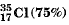and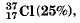calculate the average atomic mass of chlorine atom.    [CBSE 2015]
Ans.
This is calculated as under: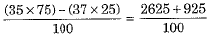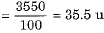Q.11. Define the term isobar with one example.    [CBSE 2015]
Ans.
Isobars are atoms of different elements having the same mass number but different atomic numbers.

Q.12. (a) Calculate the average atomic mass of chlorine if it exists commonly in two isotopes.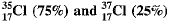(b) Write the main drawback of Rutherford’s model of an atom.    [CBSE 2015]
Ans.
(a) Average atomic mass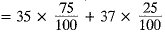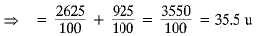(b) Draw backs of Rutherford’s Model of an Atom: Any charged particle when accelerated is expected to radiate energy. To remain in a circular orbit, the electron would need to undergo acceleration. Therefore, it would radiate energy.
The loss of energy would lead to shrinking of the orbit in size. In short time, it would hit the nucleus. Therefore, an atom cannot be expected to be stable.

Q.13. In the following table, the mass number and the atomic number of certain elements are given: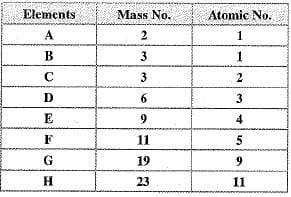(a) How many neutrons are present in ‘F'?
(b) Which atoms are isotopes of the same element?
(c) Which atom will form singly positively charged ion?
(d) Which is the atom of an inert gas?
(e) Which will form singly negatively charged ion?
(f) Which of these has 11 electrons?    [CBSE 2015]
Ans.
(a) Number of neutrons in 'F’ is equal to 11 - 5 = 6
(b) A and B are isotopes of the same element.
(с) 'D’ and ‘H’ can form singly +ve ions.
(d) Element 'C’ is inert gas.
(e) 'G’ will form singly negatively charged ion.
(f) 'H’ has 11 electrons.

Q.14. Give reasons:
(a) Mass number of an atom excludes the mass of an electron.
(b) Nucleus of an atom is charged.
(c) Alpha particle scattering experiment was possible by using gold foil only and not by foil of any other metal.    [CBSE 2015]
Ans.
(a) It is because mass of electron is negligible.
(b) Nucleus of an atom consists of positively charged protons and neutrons which do not have charge.
(c) It is because gold metal is highly malleable and very thin foil of gold foil was taken.

Q.15. What is a proton? Who discovered it?    [CBSE 2015]
Ans.
Proton is positively charged particle present in nucleus.
It was discovered by Goldstein.

Q.1. (i) Define Isotopes and Isobars with examples.
(ii) Give electronic configuration of any one pair of isotopes and isobars.    [NCT 2018]
Ans.
(i) Isotopes: Isotopes are defined as the atoms of the same element having the same atomic number but different mass numbers. For example, protium 11H and deuterium 21H.
Isobars: Atoms of different elements having same mass number but with different atomic numbers are called isobars. For example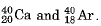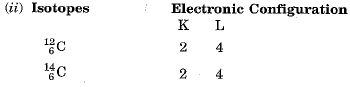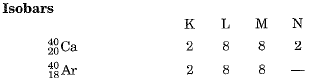Q.2. Give reasons for the following:
(а) Isotopes of an element are chemically similar.
(b) An atom is electrically neutral.
(c) Noble gases show least reactivity.
(d) Ions are more stable than atoms.
(e) Na+ has completely filled K and L shells.    [CBSE 2016]
Ans.
(a) Chemical properties of an element depend upon the atomic number. All the isotopes of an element have the same atomic number and therefore are chemically similar.
(b) An atom has the equal number of positively charged protons and negatively charged electrons. Therefore atom is electrically neutral.
(c) Noble gases contain 8 electrons in the outermost shell (2 in case of helium). This is the maximum capacity of the outermost shell. Hence they show least reactivity.
(d) Ions are more stable than atoms because the former have an octet structure.
(e) Electronic configuration of Na is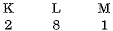Na+ is formed from Na by removing the electron from the M shell. Therefore it has the completely filled K and L shells.

Q.3. (a) Define Valency. What conclusions can be drawn about the reactivity of an atom from its valency?
(b) Why does an atom of Argon have zero valency? Explain using the electronic configuration of Argon.    [CBSE 2016]
Ans. (a) Valency is defined as the number of electrons lost or gained or shared to attain the nearest noble gas (stable) configuration. If valency is equal to 1 or 2, the element is highly reactive. If valency is equal to zero, it is not reactive. If valency equal to 3 or 4 it is less reactive.
(b) Argon has its octet complete (2, 8, 8), therefore, it cannot gain or lose or share electrons. So, its valency is equal to zero.

Q.4. The average atomic mass of a sample of an element X is 16.2 u. What are the percentage of isotope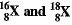in the sample.    [CBSE 2016]
(b) On the basis of Thomson’s model of an atom explain how the atom is neutral as a whole.    [CBSE 2011]
Ans. (a) Percentage of Isotopes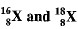Let percentage of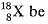'x', therefore, percentage of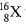will be 100 - x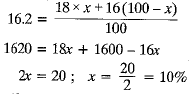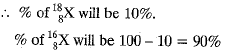(b) Positively charged particles and negative charged particles are equal, therefore, an atom is neutral on the basis of Thomson model.

Q.5. (a) A helium atom has an atomic mass of 4 u and two protons in its nucleus. How many neutrons does it have?
(b) Write down the electronic configuration of oxygen. [At. No. = 8]
(c) The atomic mass of an element S is 16.2 u. What are the percentages of isotopes of X having atomic number 8 and mass numbers 16 and 18.    [CBSE 2015]
Ans.
(a) The atomic mass of helium = 4 u
∴ No. of protons of + No. of neutrons = 4
or 2 + No. of neutrons = 4
or No. of neutrons = 4 - 2 = 2
(b) Electronic configuration of oxygen is 2, 6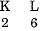(c) Let the percentage of isotope with mass no 16 = x
Then percentage of isotope with mass no. 18 = 100 - x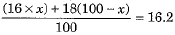or 16x + 1800 - 18x = 1620 or - 2x = 1620 - 1800
or - 2x = - 180 or x = 90
The percentage of isotope with mass number 16 = 90
Percentage of isotope with mass number 18 = 100 - 90 = 10

The document Previous Year Questions Answers: Structure of the Atom | Science Class 9 is a part of the Class 9 Course Science Class 9.
All you need of Class 9 at this link: Class 9

## Science Class 9

66 videos|352 docs|97 tests

## FAQs on Previous Year Questions Answers: Structure of the Atom - Science Class 9

 1. What is the structure of an atom?Ans. The structure of an atom consists of a nucleus at the center, which contains protons and neutrons. Around the nucleus, there are electrons that orbit in specific energy levels or shells.
 2. How do protons and neutrons differ from each other?Ans. Protons are positively charged particles found in the nucleus of an atom, while neutrons are neutral particles also present in the nucleus. Protons determine the atomic number of an element, while neutrons contribute to the mass of the atom.
 3. What are energy levels or shells in an atom?Ans. Energy levels or shells in an atom are designated regions where electrons can be found. These shells are labeled as the first, second, third, and so on, with the first shell closest to the nucleus. Each shell can hold a specific number of electrons.
 4. How are electrons distributed in an atom's energy levels?Ans. Electrons are distributed in an atom's energy levels according to the Aufbau principle, which states that electrons occupy the lowest energy level first before filling higher energy levels. The maximum number of electrons in each energy level can be calculated using the 2n^2 formula.
 5. What is the significance of the atomic number in an atom?Ans. The atomic number of an atom represents the number of protons in its nucleus. It determines the element's identity and its position in the periodic table. The number of electrons in a neutral atom is also equal to the atomic number.

## Science Class 9

66 videos|352 docs|97 testsExplore Courses for Class 9 examSignup to see your scores go up within 7 days! Learn & Practice with 1000+ FREE Notes, Videos & Tests.
10M+ students study on EduRev
Track your progress, build streaks, highlight & save important lessons and more!
Related Searches

,

,

,

,

,

,

,

,

,

,

,

,

,

,

,

,

,

,

,

,

,

;